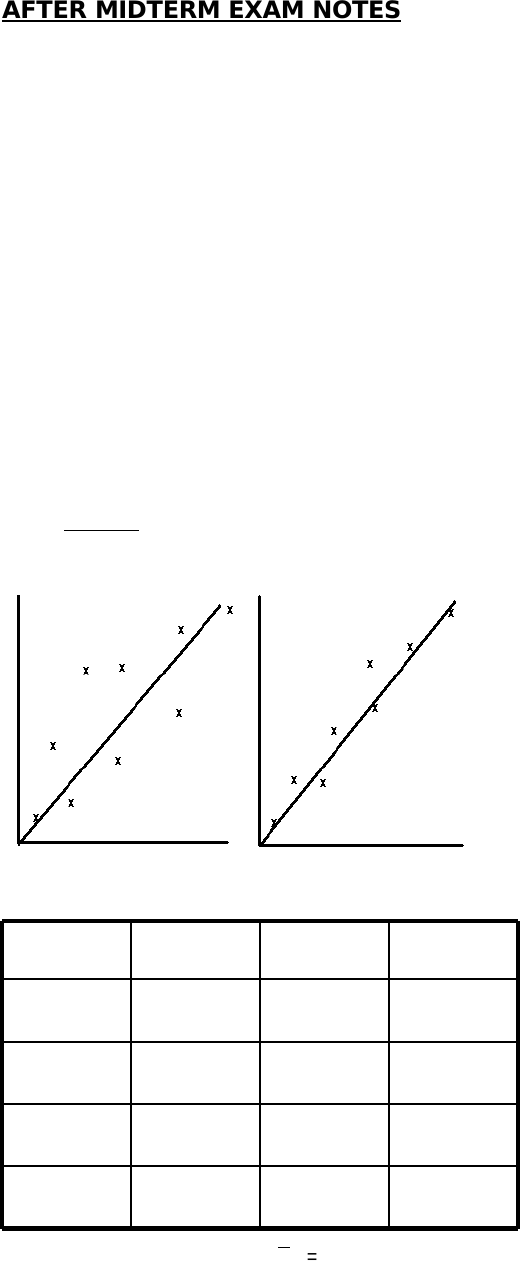Study Guides (390,000)
CA (150,000)
UTSC (10,000)
Psychology (2,000)
Final

PSYB07H3 Study Guide - Final Guide: Linear Regression, Bors, Standard Deviation

Department
Psychology
Course Code
PSYB07H3
Professor
Douglas Bors
Study Guide
Final

Page:
of 4AFTER MIDTERM EXAM NOTES
PEARSON-PRODUCT MOMENT CORRELATION COEFFICIENT (r)
-tells us the strength of the relationship between x and y
-the average product of z-scores same thing as z scores
-correlation coefficients are the covariance when there are standard
variables standardize x but leave y and its use get a measure in y
units
COVxy = a measure of the relation between x and y the covariance
standardized by the standard deviation of x and y
-to standardize , we divide the covariance by the size of the standard
deviations.
-g
iven that the maximum value of the covariance is plus or minus the
product of the variance of x and the variance of y, it follows that the
limits on the correlation coefficient are +1.0 or – 1.0
-the correlation coefficient is not an unbiased esimator
Example:
rC O V
s s
x y
x y
=
41347
01116
11205
11014
4943
x
s
y
y
=
=
1 1
1 5 8.
x- y-
If the regression coefficient is computed
the slopes can be he same but the
correlation is different i.e. the second
scatterplot has more noise.
1.
2.
3.
-expected value of r is not (rho --> Greek letter), then we correct for itρ
-correlation cannot = 1 because there will be many variables that affect
(influence) relationship of behaviour you’re trying to predict
r2 = that proportion of the variance is y that is shared (accounted for) by
x. Sometimes called “coefficient of determination
-therefore, r = 0.9 and r2 = 0.81 or x account for 81% of the variance in
y (doesn’t mean x CAUSES y to chance…it covaries
-i.e. r =0.2 thus r2 = 0.04 or 4%
-i.e. r = 0.4 thus r2 =0.16 or 16%
-if our r is g times as large as a second r, then the proportion of the
variance associated with the first r will be g(squared) times as great as
that associated with the second.
-the chance of a zero slope is slcose to zero
-you must ask how reliable is the relationship between x and y
Factors Affecting r
-correlation tells us about the relationship between the variance of x and
variance of y and what other factors affect y
1. Range Restrictions
x
s
x
x
=
=
5
1 5 8.
C O V
x y
=
2 2 5.
rC O V
s s
x y
x y
=
r
r
=
=
2 2 5
1 5 8 1 5 8
0 9
.
( . ) ( . )
.
rr N
N
= − −
11 1
2
2
( ) ( )
( )
r
a d j
= − −
11 8 1 1
2
( . ) ( 5 )
( 5 )
= .75
-you get a circle which can show that there is no relationship between x
and y (when there might be)
2. Outliers
-leads to a big z-score can create illusion of a strong/weak correlation
when there isn’t
3. Heterogeneous Subsamples
-blue: strong moderate correlation
-green strong correlation
-put blue and green together get a weak relationship weak
correlation
-indicates that you shouldn’t put two sub groups together, can be
Whole Part Correlation produces a bias
-this is where the score for variable x contributes to the score of variable
y produces a positive bias in r
-again, correlation does not imply causality variables may be
accidentally related or both may be related to a third variable or thy
may influence each other
i.e. the price of petroleum is correlated with Bors’ age but it doesn’t
mean the price is going up as Bors ages or because Bors ages it is
accidentally correlated
-what is more informative: the slope of the regression line or the
correlation coefficient? they both answer different things